# Relabel-to-front Algorithm

The relabel-to-front algorithm is used to find the maximum flow in the network. The relabel-to-front algorithm is more efficient than the generic push-relabel method. In the push-relabel method, we can apply the basic operations of push and relabel in any order. The relabel-to-front algorithm chooses the order carefully and manages the network data structures efficiently.

First, we need to understand the basic operations i.e. push and relabel:
Each vertex in the network has 2 variables associated with it which are height variable(h) and excess flow(e).

1. Push: If a vertex has excess flow and there is an adjacent node with lower height (in the residual graph) then we push flow from the vertex to the lower height node.
2. Relabel: If a vertex has excess flow and no adjacent node with lower height are available then we use relabel operation to increase the height of vertex so that it can perform push operation.

The relabel-to-front algorithm maintains a list of vertices in the network. It starts from the beginning of the list and repeatedly selects an overflowing vertex u and performs discharge operation on it.
Discharge operation is performing push and relabel operation until vertex u has no positive excess flow(e)
If a vertex is relabeled, it is moved to the front of the list and algorithm scans again.

Algorithm:

• Initialize the preflow and heights to the same values as in the generic push-relabel algorithm.
• Initialize list L which contains all vertices except source and sink.
• Initialize current pointer of each vertex u to the first vertex in u’s neighbour list N. The neighbour list N contains those vertices for which there is a residual edge.
• While algorithm reaches the end of list L.
• Select the vertex u from list L and perform discharge operation.
• If u was relabeled by discharge then move u to the front of the list.
• If u was moved to the front of the list, the vertex in the next iteration is the one following u in its new position in the list.

Example:
Consider the given flow network.On the right is shown the initial list L=(B, C) where initially u=B.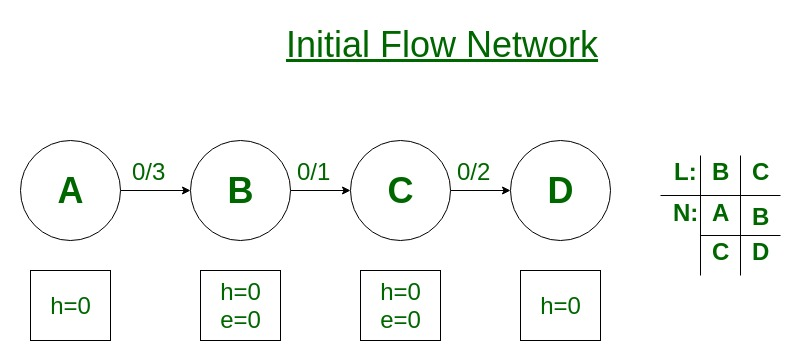After the preflow initialization operation.Under each vertex in list L is its neighbor list N with the current neighbor circled.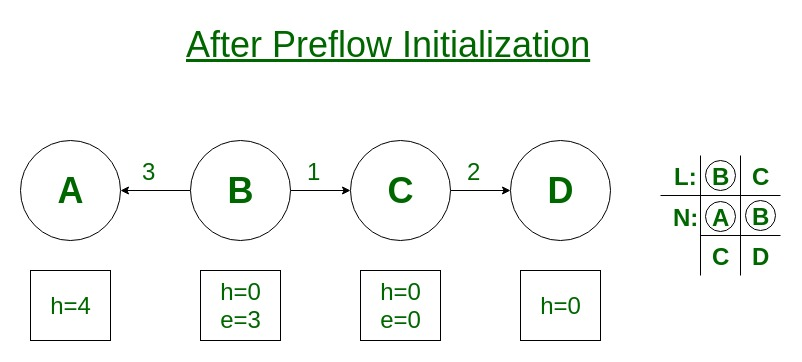Vertex B undergoes discharge operation as it has excess flow 3 (e=3).Vertex B has no node with lower height thus it performs relabel operation (h=1) and pushes flow 1 to vertex C.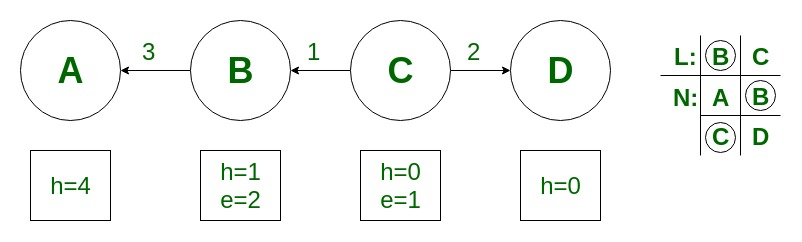Vertex B still has excess flow 2(e=2) thus it performs relabel operation (h=5)and pushes flow 2 to vertex A.Since vertex B is relabeled it remains in the front of the list. Now vertex C undergoes discharge operation as it has excess flow 1(e=1).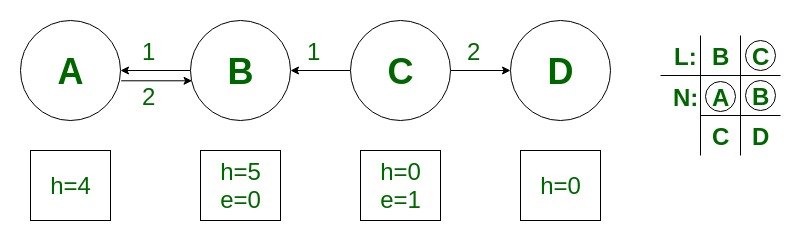Vertex C performs relabel operation(h=1) and pushes flow 1 to node D.Since vertex C performed relabel operation it is moved to the front of the list.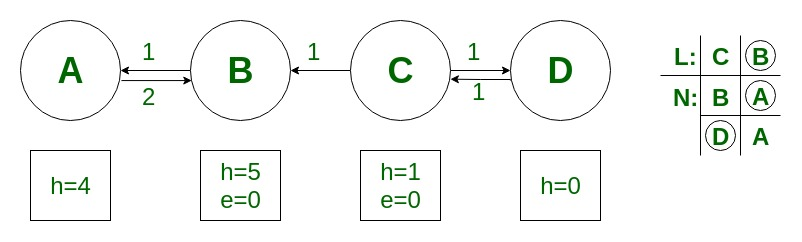Vertex B now follows vertex C in L but B has no excess flow. The RELABEL-TO-FRONT has reached the end of list L and terminates. There are no overflowing vertices, thus the preflow is a maximum flow. Here max flow is 1.

Time Complexity: Runs in O(V3) time on network G = (V, E). Thus it is more efficient than generic push-relabel algorithm which runs in O(V2E) time.

GeeksforGeeks has prepared a complete interview preparation course with premium videos, theory, practice problems, TA support and many more features. Please refer Placement 100 for details

My Personal Notes arrow_drop_upCheck out this Author's contributed articles.

If you like GeeksforGeeks and would like to contribute, you can also write an article using contribute.geeksforgeeks.org or mail your article to contribute@geeksforgeeks.org. See your article appearing on the GeeksforGeeks main page and help other Geeks.

Please Improve this article if you find anything incorrect by clicking on the "Improve Article" button below.